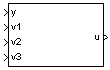# 3D Controller [A(v),B(v),C(v),D(v)]

Implement gain-scheduled state-space controller depending on three scheduling parameters

•Libraries:
Aerospace Blockset / GNC / Control

## Description

The 3D Controller [A(v),B(v),C(v),D(v)] block implements a gain-scheduled state-space controller as described in Algorithms.

The output from this block is the actuator demand, which you can input to an actuator block.

## Limitations

If the scheduling parameter inputs to the block go out of range, they are clipped. The state-space matrices are not interpolated out of range.

## Ports

### Input

expand all

Aircraft measurements, specified as a vector.

Data Types: `double`

First scheduling variable, specified as a vector, that conforms to the dimensions of the state-space matrices.

Data Types: `double`

Second scheduling variable, specified as a vector, that conforms to the dimensions of the state-space matrices.

Data Types: `double`

Second scheduling variable, specified as a vector, that conforms to the dimensions of the state-space matrices.

Data Types: `double`

### Output

expand all

Actuator demands, specified as a vector.

Data Types: `double`

## Parameters

expand all

A-matrix of the state-space implementation. In the case of 3-D scheduling, the A-matrix should have five dimensions, the last three corresponding to scheduling variables v1, v2, and v3. For example, if the A-matrix corresponding to the first entry of v1, the first entry of v2, and the first entry of v3 is the identity matrix, then `A(:,:,1,1,1) = [1 0;0 1];`.

#### Programmatic Use

 Block Parameter: `A` Type: character vector Values: vector Default: `'A'`

B-matrix of the state-space implementation. In the case of 3-D scheduling, the B-matrix should have five dimensions, the last three corresponding to scheduling variables v1, v2, and v3. For example, if the B-matrix corresponding to the first entry of v1, the first entry of v2, and the first entry of v3 is the identity matrix, then `B(:,:,1,1,1) = [1 0;0 1];`.

#### Programmatic Use

 Block Parameter: `B` Type: character vector Values: vector Default: `'B'`

C-matrix of the state-space implementation. In the case of 3-D scheduling, the C-matrix should have five dimensions, the last three corresponding to scheduling variables v1, v2, and v3. For example, if the C-matrix corresponding to the first entry of v1, the first entry of v2, and the first entry of v3 is the identity matrix, then `C(:,:,1,1,1) = [1 0;0 1];`.

#### Programmatic Use

 Block Parameter: `C` Type: character vector Values: vector Default: `'C'`

D-matrix of the state-space implementation. In the case of 3-D scheduling, the D-matrix should have five dimensions, the last three corresponding to scheduling variables v1, v2, and v3. For example, if the D-matrix corresponding to the first entry of v1, the first entry of v2, and the first entry of v3 is the identity matrix, then `D(:,:,1,1,1) = [1 0;0 1];`.

#### Programmatic Use

 Block Parameter: `D` Type: character vector Values: vector Default: `'D'`

Vector of the breakpoints for the first scheduling variable. The length of v1 should be same as the size of the third dimension of A, B, C, and D.

#### Programmatic Use

 Block Parameter: `AoA_vec` Type: character vector Values: vector Default: `'v1_vec'`

Vector of the breakpoints for the second scheduling variable. The length of v2 should be same as the size of the fourth dimension of A, B, C, and D.

#### Programmatic Use

 Block Parameter: `AoS_vec` Type: character vector Values: vector Default: `'v2_vec'`

Vector of the breakpoints for the third scheduling variable. The length of v3 should be same as the size of the fifth dimension of A, B, C, and D.

#### Programmatic Use

 Block Parameter: `Mach_vec` Type: character vector Values: vector Default: `'v3_vec'`

Vector of initial states for the controller, i.e., initial values for the state vector, x. It should have length equal to the size of the first dimension of A.

#### Programmatic Use

 Block Parameter: `x_initial` Type: character vector Values: vector Default: `'0'`

## Algorithms

The block implements a gain-scheduled state-space controller as defined by this equation:

`$\begin{array}{l}\stackrel{˙}{x}=A\left(v\right)x+B\left(v\right)y\\ u=C\left(v\right)x+D\left(v\right)y\end{array}$`

where v is a vector of parameters over which A, B, C, and D are defined. This type of controller scheduling assumes that the matrices A, B, C, and D vary smoothly as a function of v, which is often the case in aerospace applications.

## Version History

Introduced before R2006a